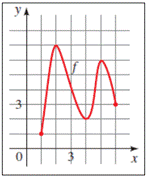# To fill in the gaps : with reference to the provided graph### Precalculus: Mathematics for Calcu...

6th Edition
Stewart + 5 others
Publisher: Cengage Learning
ISBN: 9780840068071### Precalculus: Mathematics for Calcu...

6th Edition
Stewart + 5 others
Publisher: Cengage Learning
ISBN: 9780840068071

#### Solutions

Chapter 2.3, Problem 1E
To determine

## To fill in the gaps: with reference to the provided graph

Expert Solution

To find the value of a function f (a) from the graph of f , we find the height of the graph above the x -axis at x = a. From the graph of x , we see that f (3) = 4.

### Explanation of Solution

Given information: To find the value of a function f (a) from the graph of f , we find the height of the graph above the x -axis at x = _______ . From the graph of x , we see that f (3) = ______.To find the value of a function f (a) from the graph of f , we find the height of the graph above the x -axis at x = a .

From the graph, f (3) = 4 , since, the height of the graph at x = 3 is 4 units.

### Have a homework question?

Subscribe to bartleby learn! Ask subject matter experts 30 homework questions each month. Plus, you’ll have access to millions of step-by-step textbook answers!